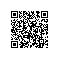# 二维数组的查找及向函数传递二维数组问题#include <stdio.h>

int find_value(int a[], int value, int x, int y)//(x,y)表示右上角坐标
{
if ((x >= 0) && (y < 4))
{
if (a[x][y] ==  value)
return 1;
else if (a[x][y] > value)
return find_value(a, value, x-1, y);
else
return find_value(a, value, x, y+1);
}

return 0;
}

int main()
{
int a = {{1, 2, 8, 9}, {2, 4, 9, 12}, {4, 7, 10, 13}, {6, 8, 11, 15}};
int val;
scanf("%d", &val);
if(find_value(a, val, 3, 0))
printf("Yes, find it\n");
else
printf("No, not find it\n");

return 0;
}

1.二维数组的定义——第二位长度必须指明

我们知道一位数组定义可以不指名元素总的个数，例如int a[] = {1, 2, 3}，编译器会自动赋值长度为3；对于字符char a[] = {'a', 'b', 'c'}，自动赋值为4,（因为字符数组末尾有个默认‘\0’字符）

2.向函数中传递二维数组

方法一：形参给出第二维大小

#include <stdio.h>
void Find(int a[])   //此处必须指明第二维的大小
{
int i, j;
for(i=0; i<3; i++)
for (j=0; j<3; j++)
printf("%d\n", a[i][j]);
}
int main()
{
int a = {{1, 2, 3}, {4, 5, 6}, {7, 8, 9}};
Find(a);

return 0;
}

#include <stdio.h>
void Find(int (*a))
{
int i, j;
for(i=0; i<3; i++)
for (j=0; j<3; j++)
printf("%d\n", a[i][j]);
}
int main()
{
int
a = {{1, 2, 3}, {4, 5, 6}, {7, 8, 9}}
; Find(a); return 0; }

#include <stdio.h>
void Find(int (*a))
{
int i, j;
printf("%d\n", *a);
}
int main()
{
int a = {{1, 2, 3}, {4, 5, 6}, {7, 8, 9}};
Find(a);

return 0;
}

3.sizeof

sizeof(type)   //类型

#include <stdio.h>
int main()
{
//bool aa;
char a;
int c;

int *i;
char *j;

printf("int_c:%lu**type_int:%lu\n", sizeof(c), sizeof(int));
printf("char_a:%lu**type_char:%lu\n", sizeof(char), sizeof(char));

printf("int *i:%lu**%lu\n", sizeof(i), sizeof(int));
printf("char *j:%lu**%lu\n", sizeof(j), sizeof(int));

return 0;
}#include <stdio.h>
int main()
{
int array[] = {1, 2, 3};

printf("array:%lu  ###*array:%lu  ###array+0:%lu\n", sizeof(array), sizeof(*array), sizeof(array+0));
printf("size1:%lu\n", sizeof(array) / sizeof(*array));
printf("size2:%lu\n", sizeof(array) / sizeof(array));

return 0;
}下面看二位数组

#include <stdio.h>
int main()
{
int array[] = {1, 2, 3, 4, 5};

printf("array:%lu  ###*array:%lu  ###array+0:%lu  ##**array:%lu\n", sizeof(array), sizeof(*array), sizeof(array+0), sizeof(**array));
printf("size1:%lu\n", sizeof(array) / sizeof(*array));
printf("size2:%lu\n", sizeof(array) / sizeof(array));

return 0;
}4.二维数组传给函数当一维数组用——利用下标转换成一维数组的下标

#include <stdio.h>
void Find(int *a, int num)
{
int i = 0;
for(; i< num; i++)
printf("%d\n", a[i]);
}
int main()
{
int a = {{1, 2, 3}, {4, 5, 6}, {7, 8, 9}};
Find((int*)a, 9);

return 0;
}

*********************好了，修改下思路三***********************

#include <stdio.h>

int  find_value(int *a, int val, int rows, int cols) //val是待查元素。rows是二维数组行数，cols是列数
{
int row = 0;
int col = cols-1;
while(row < rows && col >= 0)
{
if (a[row * cols + col] ==  val)
return 1;
else if (a[row * cols + col] > val)
col--;
else
row++;
}

return 0;
}

int main()
{
int a[] = {1, 2, 8, 9, 2, 4, 9, 12, 4, 7, 10, 13,6, 8, 11, 15};
int val;
scanf("%d", &val);
if(find_value((int*)a, val, 4, 4))
printf("Yes, find it\n");
else
printf("No, not find it\n");

return 0;
}使用钉钉扫一扫加入圈子
+ 订阅